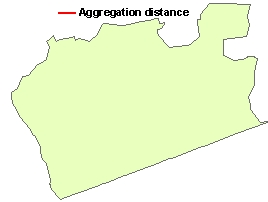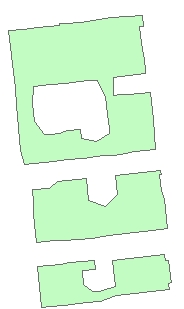Aggregate Polygons

Go to ToolBox Implementation

Combines the polygons that are within the user specified distance into new polygons. Can be used also to generalize buildings.

Inputs:

• A polygon feature class
• Aggregation distance. The polygons that are closer to each other than this distance will be combined
• Minimum area of the holes to be preserved - all holes with area less than this tolerance will be removed.

Outputs:

• New  polygon feature class

Notes:

• The new feature class will not have any attributes. The Transfer Attributes function can be used to get summarized attributes from the original polygons.
• The Aggregation distance and the Minimum area of holes should be specified in the units of the spatial reference of the input feature class
• If no Minimum area of holes is specified only the holes with area  smaller than 2 x Aggregation distance x Aggregation distance will be removed
• The function is CPU and RAM intensive. Using it on large datasets might need long processing time.

Examples:

• General polygons
 Input Dataset Result (No Minimum area of holes specified) Result (Minimum area of holes specified)• Buildings
 Input Dataset Aggregate distance = 1 meter Aggregate distance = 5 meters Aggregate distance = 10 m Aggregate distance = 20 mCommand line syntax

ET_GPAggregatePolygons <input_dataset> <out_feature_class> <Aggregate_tolerance> <area_tolerance>

Parameters

Expression Explanation
<input_dataset> A Polyline feature class or feature layer
<out_feature_class> A String - the full name of the output feature class (A feature class with the same full name should not exist)
<Aggregate_tolerance> A Double representing  the aggregation distance. The polygons that are closer to each other than this distance will be combined
<area_tolerance> A Double representing  the minimum area of holes to be preserved. All holes with area less than this tolerance will be removed.

Scripting syntax

ET_GPAggregatePolygons (input_dataset, out_feature_class, Aggregate_tolerance,area_tolerance)

See the explanations above:
<> - required parameter
{} - optional parameter

Example Python script:

 import arcpyarcpy.ImportToolbox("C:/Program Files/ET SpatialTechniques/ET GeoWizards 11.0 for ArcGIS 10.1/ET GeoWizards.tbx")input_dataset = "C:\\data\\pg1.shp" result = "C:\\data\\fgdb_test.gdb\\aggregated"arcpy.gp.toolbox = "C:/Program Files/ET SpatialTechniques/ET GeoWizards 11.0 for ArcGIS 10.1/ET GeoWizards.tbx"arcpy.ET_GPAggregatePolygons(input_dataset,  result, 10.00, 1000.00)